# Find a cone in R2 that is not convex, Prove that a subset X of Rr is a convex cone if and only if x,y eX implies that X...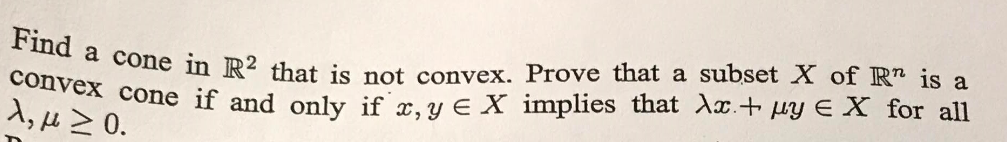Find a cone in R2 that is not convex, Prove that a subset X of Rr is a convex cone if and only if x,y eX implies that Xx+ py E X for all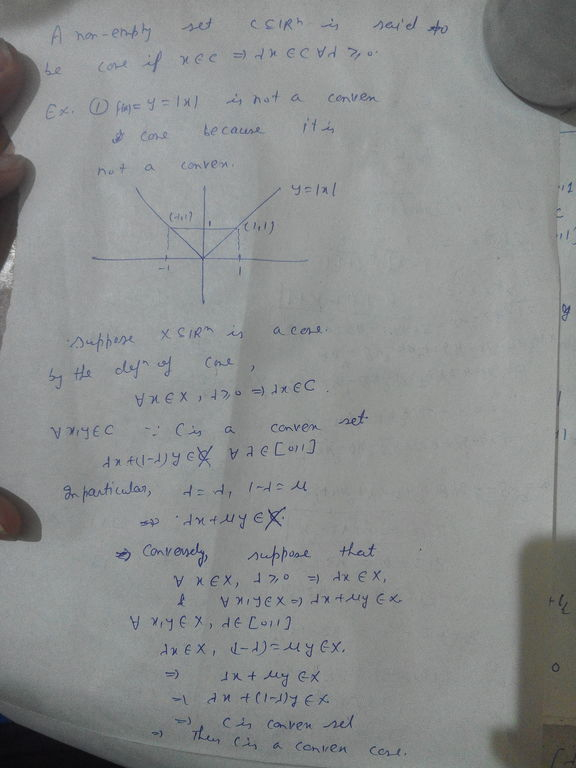##### Add Answer of: Find a cone in R2 that is not convex, Prove that a subset X of Rr is a convex cone if and only if x,y eX implies that X...
Similar Homework Help Questions
• ### 1. (2 points) Let (X, 1 1) be a Banach space, K C X be a closed and convex subset and a eX Suppos...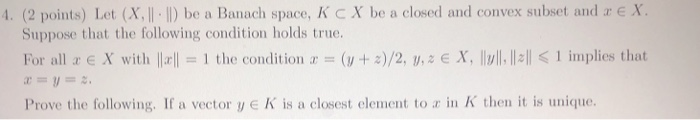1. (2 points) Let (X, 1 1) be a Banach space, K C X be a closed and convex subset and a eX Suppose that the following condition holds true. For all x E X with | = 1 the condition a-(w+ z)/2, U, z e X, llull, ll:l < i implies that Prove the following. If a vector y K is a closest element to a in K then it is unique. 1. (2 points) Let (X, 1 1)...

• ### Prove that there is no continuous bijection from the unit circle S1 = y21 onto any subset of R. (x,y) E R2 Prove that there is no continuous bijection from the unit circle S1 = y21 onto any subs...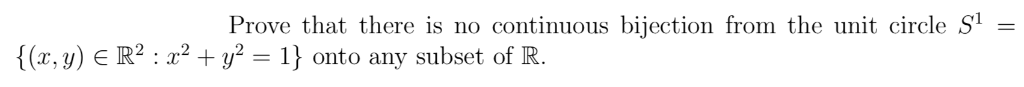Prove that there is no continuous bijection from the unit circle S1 = y21 onto any subset of R. (x,y) E R2 Prove that there is no continuous bijection from the unit circle S1 = y21 onto any subset of R. (x,y) E R2

• ### Example: Let x, y ∈ Rn, where n ∈ N. The line segment joining x to y is the subset {(1 − t)x + ty...

Example: Let x, y ∈ Rn, where n ∈ N. The line segment joining x to y is the subset {(1 − t)x + ty : 0 ≤ t ≤ 1 } of R n . A subset A of Rn, where n ∈ N, is called convex if it contains the line segment joining any two of its points. It is easy to check that any convex set is path-connected. (a) Let f : X → Y be an...

• ### (2) (a) Prove that there is a C1 map u: E → R2 defined in a neighborhood E C R2 of the point (1,0) such that (b) Find Du(x) for x є E. (c) Prove that there is a C map v G R2 defined in a neighborhood...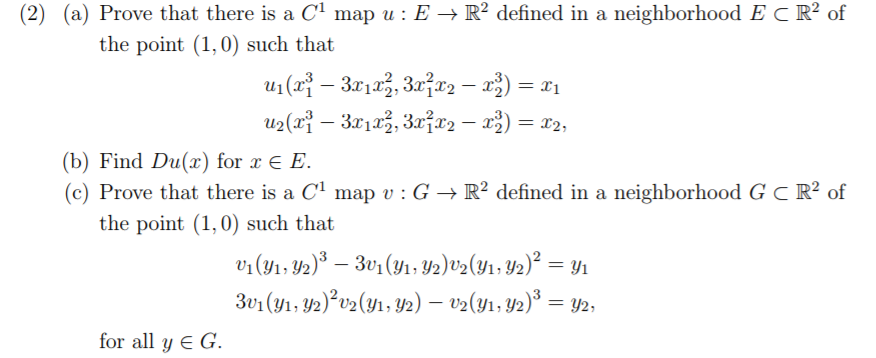(2) (a) Prove that there is a C1 map u: E → R2 defined in a neighborhood E C R2 of the point (1,0) such that (b) Find Du(x) for x є E. (c) Prove that there is a C map v G R2 defined in a neighborhood G C R2 of the point (1,0) such that for all y G. (2) (a) Prove that there is a C1 map u: E → R2 defined in a neighborhood E C...

• ### 2. Consider the following transformations of R2 Tİ (z, y) (-r, y), T3(x, y) (z, _y), T,(zw) (y, x). Show that, for any j 1,2,3, a subset A C R2 is a Jordan region if and only if T,(A) is a Jordan...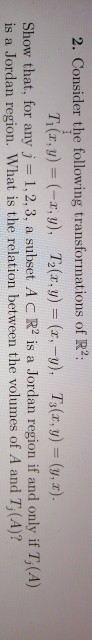2. Consider the following transformations of R2 Tİ (z, y) (-r, y), T3(x, y) (z, _y), T,(zw) (y, x). Show that, for any j 1,2,3, a subset A C R2 is a Jordan region if and only if T,(A) is a Jordan region. What is the relation between the volumes of A and T, (A)? 2. Consider the following transformations of R2 Tİ (z, y) (-r, y), T3(x, y) (z, _y), T,(zw) (y, x). Show that, for any j 1,2,3,...

• ### (2) (a) Prove that there is a C1 map u : E → R-defined in a neighborhood E c R2 of the point (1,0) such that (b) Find u'(x) for x E E (c) Prove that there is a Cl map : G → R2 defined in a neighb...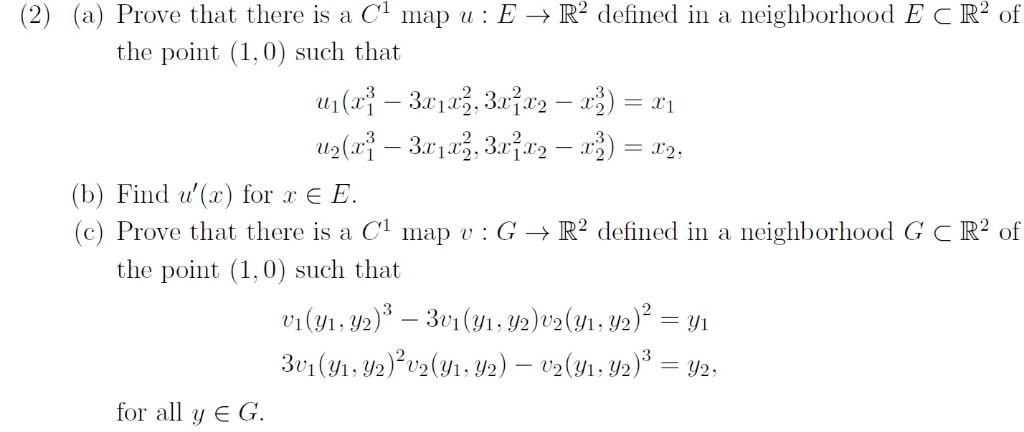(2) (a) Prove that there is a C1 map u : E → R-defined in a neighborhood E c R2 of the point (1,0) such that (b) Find u'(x) for x E E (c) Prove that there is a Cl map : G → R2 defined in a neighborhood G C R2 of the point (1,0) such that for all y EG (2) (a) Prove that there is a C1 map u : E → R-defined in a neighborhood E...

• ### 1(a) Let f : R2 → R b constant M > 0 such that livf(x,y)|| (0.0)-0. Assume that there exists a e continuously differentiable, with Mv/r2 + уг, for all (z. y) E R2 If(x,y)| 〈 M(x2 + y2)· for all (...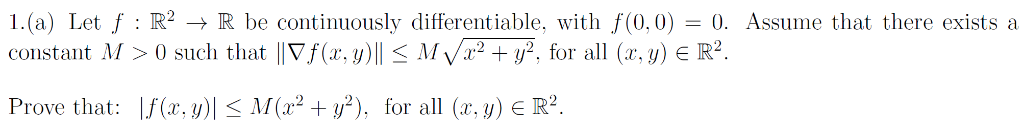1(a) Let f : R2 → R b constant M > 0 such that livf(x,y)|| (0.0)-0. Assume that there exists a e continuously differentiable, with Mv/r2 + уг, for all (z. y) E R2 If(x,y)| 〈 M(x2 + y2)· for all (a·y) E R2 Prove that: 1(a) Let f : R2 → R b constant M > 0 such that livf(x,y)|| (0.0)-0. Assume that there exists a e continuously differentiable, with Mv/r2 + уг, for all (z. y) E R2...

• ### Let U be an open subset of R. Let f: U C Rn → Rm. (a) Prove that f is continuously differentiable if and only if for ea...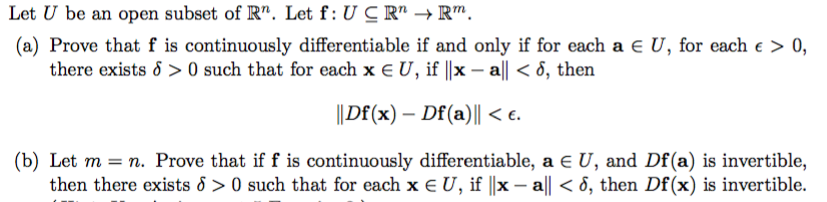Let U be an open subset of R. Let f: U C Rn → Rm. (a) Prove that f is continuously differentiable if and only if for each a є U, for each E > 0, there exists δ > 0 such that for each x E U, if IIx-all < δ, then llDf(x)-Df(a) ll < ε. (b) Let m n. Prove that if f is continuously differentiable, a E U, and Df (a) is invertible, then there exists δ...

• ### Assume f : R" → R is twice continuously differentiable. Prove that the following are equivalent: ...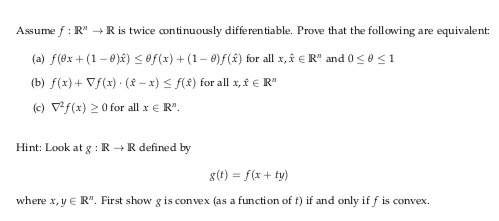Assume f : R" → R is twice continuously differentiable. Prove that the following are equivalent: (a) f(ex + (1-8)ì) < ef(x) + (1-8)/(x) for all x, x E Rn and 0 < θ < 1 (b) f(x)+ /f(x) . (x-x) -f(r) for all x,x E R" (c) f(x) > 0 for all x E R" Hint: Look at : RRdefine by gt) f(x + ty) where x, y E R. First show g is convex (as a function of...

• ### 1. Prove that the function f: X → Y is injective if and only if it...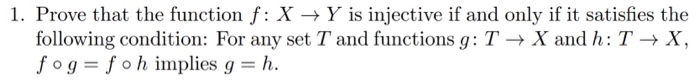1. Prove that the function f: X → Y is injective if and only if it satisfies the following condition: For any set T and functions g: T → X and h : T → X, o g = f o h implies g = h.

Free Homework App